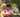### Frog River One

January 17, 2020

Frog River One

A small frog wants to get to the other side of a river. The frog is initially located on one bank of the river (position 0) and wants to get to the opposite bank (position X+1). Leaves fall from a tree onto the surface of the river.

You are given an array A consisting of N integers representing the falling leaves. A[K] represents the position where one leaf falls at time K, measured in seconds. The goal is to find the earliest time when the frog can jump to the other side of the river.

The frog can cross only when leaves appear at every position across the river from 1 to X (that is, we want to find the earliest moment when all the positions from 1 to X are covered by leaves). You may assume that the speed of the current in the river is negligibly small, i.e. the leaves do not change their positions once they fall in the river.

For example, you are given integer X = 5 and array A such that: A = 1
A = 3
A = 1
A = 4
A = 2
A = 3
A = 5
A = 4 In second 6, a leaf falls into position 5. This is the earliest time when leaves appear in every position across the river.

Write a function:
function solution(X, A);
that, given a non-empty array A consisting of N integers and integer X, returns the earliest time when the frog can jump to the other side of the river.

If the frog is never able to jump to the other side of the river, the function should return −1.

For example, given X = 5 and array A such that: A = 1
A = 3
A = 1
A = 4
A = 2
A = 3
A = 5
A = 4
the function should return 6, as explained above.

Write an efficient algorithm for the following assumptions:
• N and X are integers within the range [1..100,000];
• each element of array A is an integer within the range [1..X].

Use Set to store unique values from A[]. When the size equals to X, means the leaves have covered all positions from 1 to X. So the frog can get to the position X+1.

``````function solution(X, A) {
// write your code in JavaScript (Node.js 8.9.4)
let holdValues = new Set()
for(i=0;i<A.length;i++) {
holdValues.add(A[i]); // stores unique values only
if(holdValues.size == X)return i;
}

return -1; // leaves didn't cover all positions 1..X
}``````Posted by Tai Lu ( 呂台生 ) who's building useful things.You should follow him on Twitter